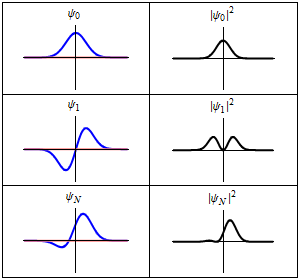# A World Of Pure Imagination

In Mathematics by Brian Koberlein3 Comments

In science, physical quantities are represented by numbers. A block has a mass of 42 kilograms, a car moves at 25 meters per second, or a star is 142 light years away. In mathematics these are known as real numbers. One of the basic properties of real numbers is that any real number multiplied by itself is a positive number. For example, 3 x 3 is 9, and (-4) x (-4) is 16. But imagine a number where its product is actually negative. For example, what if some number i works so that i x i is -1. Such a number is known as an imaginary number. The term imaginary number often gives the impression that these numbers are merely mathematical abstractions, but when it comes to the physical universe, imaginary numbers are quite real.

Complex numbers on a plane.

While any two imaginary numbers will always yield a negative number, when you add imaginary numbers to real ones, things start to get interesting. For example, 2 + 4 = 6 and 3i + 2i = 5i, but 2 + 3i can’t be simplified to a real or imaginary number. Such combinations are known as complex numbers, and they have a real number part and an imaginary number part. Because of this, complex numbers share a similarity with the vectors we talked about last time. Just as real numbers can be imagined as points on a number line, complex numbers can be imagined as points on a number plane. Any point on this complex plane can be represented by a distance (magnitude) and direction from your origin.

This makes complex numbers extremely useful when describing certain physical phenomena. For example, light is an electromagnetic wave that has properties such as amplitude and wavelength, but it also has an orientation known as its polarization. Complex numbers can describe light wave as it travels through space can be represented as a complex number, where the real and imaginary parts tell us the orientation of the wave. While we could describe the polarization with real numbers, complex numbers can do it much more simply and elegantly. The same is true with many other physical processes, such as oscillating currents in a circuit, or the vibration of a bridge under stress.

Given the oddness of imaginary numbers, it’s still tempting to think of them as merely a useful mathematical tool. But it turns out there are some physical processes where imaginary numbers are not just useful, they are downright required. Take, for example, the strange world of quantum theory.The real (blue) and imaginary (red) parts of a wavefunction tell us where an object is likely to be.

The physics of quantum objects is difficult to wrap our heads around, and there are lots of interpretations to help us understand. One of the most popular versions is known as the Copenhagen interpretation. In this view, a quantum object is described by a wavefunction. Just as the function of motion for a baseball can be determined by Newton’s laws of motion, the wavefunction of an object can be determined by the Schrödinger equation. But there’s a fundamental difference. The wavefunction by itself doesn’t tell you what a quantum object is doing. Instead, if you multiply the wavefunction by itself (technically by its complex conjugate) then you get a real function that tells you what the object is likely to do. Mathematically, a solution to Schrödinger’s equation contains imaginary numbers. It has to in order to accurately describe the way a quantum object behaves.

So rather than simply being abstract mathematics, or merely a useful tool, the very fabric of matter depends upon imaginary numbers. At its heart, our world is (mathematically) an imaginary world.

Next time: Complex numbers are useful because of the relationship between real and imaginary numbers. It turns out that in mathematics the relationships between numbers is what really matters. As we’ve come to understand this, we’ve also come to realized that mathematical relationships are also at the very heart of the cosmos.

1.2.3.